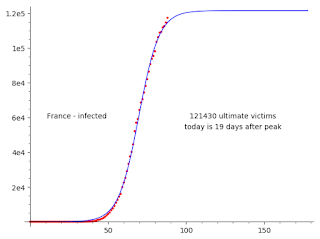## Thursday, April 23, 2020

### SIR with SageVery simple implementation of the SIR epidemic model using Sage
mathematical framework.
 ```# Infected IInit = 1 # Susceptible SInit = N - IInit # Resistant RInit = 0 # Transmission rate betaInit = 0.5 # Transmission rate attenuation delta = 0.01 # Recovery rate gamma = 0.1 # Population size N = 10000 # End time tMax = 100 # Number of points points = 1000 # Standard SIR model def ODE_RHS(t, Y): (S, I, R, beta) = Y dS = - beta * S * I / N dI = beta * S * I / N - gamma * I dR = gamma * I dbeta = - beta * delta return (dS, dI, dR, dbeta) # Set up numerical solution of ODE solver = ode_solver(function = ODE_RHS, y_0 = (SInit, IInit, RInit, betaInit), t_span = (0, tMax), algorithm = 'rk8pd') # Numerically solve solver.ode_solve(num_points = points) # Plot solution show(plot(solver.interpolate_solution(i = 0), 0, tMax, legend_label = 'S(t)', color = 'green') + plot(solver.interpolate_solution(i = 1), 0, tMax, legend_label = 'I(t)', color = 'red') + plot(solver.interpolate_solution(i = 2), 0, tMax, legend_label = 'R(t)', color = 'blue')) ```

Result:

## Wednesday, April 22, 2020

### Sigmoidal Fit

Interesting.
When I do extremely naive sigmoidal fit to the evolution of the number of virus victims in France, I get about the same prevision as prof.Raoult - that the epidemie will end very soon.
This can mean two things:
- Either I'm as clever as prof.Raoult.
- Or the other way.## Thursday, April 9, 2020

### We Will Win

We will win. When we concentrate, we can do almost anything - land on the Moon, control nuclear power, destroy the virus. And we will do it much faster than ever in history - thanks to the globalization.

The economy will reboot faster than most expect. This is not an economical crisis. Once all is over, we will travel more than ever, we will produce more then ever. We will create a big debt - and we will pay it by the inflation. Which is not a bad thing, inflation means that the rich pay more then others.

The only real danger are those people, lobbies and activists, who want to use the problems to pass their demagogic agenda, which they have failed to pass by the democratic means. We should not let them to profit from the crises.

## Monday, April 6, 2020

### Not the end

Now this is not the end.
It is not even the beginning of the end.
But it is, perhaps, the end of the beginning.
#WeWillMeetAgain